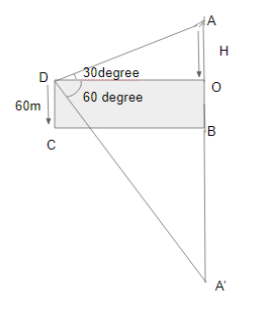QuestionAnswers

# The angle of elevation of a cloud from a point 60m/s above the surface of the water a lake is 30 degree and the angle of depression of its shadow from the same point in the water of the lake is 60 degree. Find the height of the cloud from the surface of the water.

Hint: In the given question first we will draw the diagram according to the given conditions in the question. Then according to the question, we will make equations for the given angle of elevation and angle of depression which are 30 degrees and 60 degrees respectively, and find the value of height. Thus we will get the right answer.

Complete step by step solution: Draw the diagram according to the question:Now
Let
AO=H
CD=OB=$60$m
A’B=AB=$60 + h$ ………………..$\left( 1 \right)$
Now in triangle AOD
$\tan {30^0} = \dfrac{{AO}}{{OD}} = \dfrac{H}{{OD}} \\ H = \dfrac{{OD}}{{\sqrt 3 }} \\$
Because the value of $\tan {30^0} = \dfrac{1}{{\sqrt 3 }}$
Thus
$OD = \sqrt 3 H$
Now in triangle A’OD
$\tan {60^0} = \dfrac{{OA'}}{{OD}} = \dfrac{{OB + BA'}}{{OD}} \\ \Rightarrow \sqrt 3 = \dfrac{{60 + 60 + H}}{{\sqrt 3 H}} = \dfrac{{120 + H}}{{\sqrt 3 H}} \\$
Because the value of $\tan {60^0} = \sqrt 3$
And according to equation 1
We get
A’B=AB=$60 + h$
Now
$120 + H = 3H \\ \Rightarrow 120 = 2H \\ \Rightarrow H = 60m \\$

Thus the height of the cloud from the surface of the water is $60m$.

Note: First of all draw we have to draw a system diagram for the question according to the given instruction. The angle of elevation and angle of depression is given in the question. We have to remember the actual definition of Angle of elevation and angle of depression, so that diagrams and equations can be made properly. After making an equation for both Angle of elevation and angle of depression compare them and find the height of the cloud from the surface.